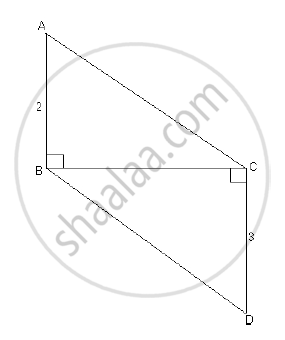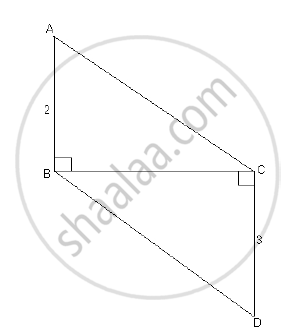# In the following figure seg AB ⊥ seg BC, seg DC ⊥ seg BC. If AB = 2 and DC = 3, find A(△ABC)/A(△DCB) - Geometry

In the following figure seg AB ⊥ seg BC, seg DC ⊥ seg BC. If AB = 2 and DC = 3, find (A(triangleABC))/(A(triangleDCB))#### Solution

In the following figure ΔABC and ΔDCB have a comman base BC.

therefore(A(triangleABC))/(A(triangleDCB))=(AB)/(DC)

(∵The ratio of areas of two triangles with the same base is equal to the ratio of their corresponding heights.)

therefore(A(triangleABC))/(A(triangleDCB))=2/3Concept: Properties of Ratios of Areas of Two Triangles
Is there an error in this question or solution?
2014-2015 (March) Set B

Share